# mini-SSE-L1-BLAS library: A fast library for SDOT,DDOT,SAXPY,DAXPY operations on x86 processor

Document version v1.01
The PDF version of this page is available here.

The mini-SSE-L1-BLAS Library is providing the basic Level 1 BLAS operations on vectors. In particular, the four functions: SDOT, DDOT, SAXPY, DAXPY were deeply hand-optimized. The library is composed of only 2 small files (a ".cpp" and a ".h" file). Inclusion of the library inside your own projects is easy. It is cross-platform. It compiles transparently on both Visual Studio .NET and GCC. A FORTRAN interface is also available. Optimization includes inline SSE and SSE2 assembly code inserted inside the C/C++ code, loop unrolling. Assembly code instructions are ordered to increase parallel execution of instructions, to ease branch prediction, to reduce dependency links between two close instructions. Software Prefetch instructions were very roughly investigated but were not included in the library. The functions are also implemented as C++ macros to remove the function calls overhead.

SDOT and DDOT operations compute dot vector products (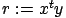with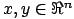). SAXPY and DAXPY operations compute(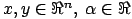). The S or D prefix on SDOT,DDOT,SAXPY,DAXPY operations means that the operations are either performed on float's (S) or on double's (D).

You can download here the mini-SSE-L1-BLAS Library (v1.03;July 15,2006).

A complete User's guide for the FORTRAN interface and for the C++ interface is given respectively in section 1.3 and section 1.4. If you are in a hurry, you can skip all the other sections and only read the user's guides (sections 1.3 and 1.4)..

This library is currently used inside my linear identification tool.

The remaining of this section is about the different code optimizations that were used for the development of the library. Section 1.2 is about experimental benchmark results. The experimental results show that the computing time can sometime be divided by four compared to a standard C implementation. The last section (1.5) gives some references and useful links.

Here is some background details about the different code optimizations that have been performed:

• As a general rule of thumb, when optimizing code you should never try to guess the best strategy based on some empirical reasoning. Always validate your choices using experimental benchmarks results only.

• SSE and SSE2 are a set of assembly-level instructions that allows to compute 4 products of float's or 2 products of double's in, essentially, the same time as 1 product using the classical set of assembly-level instructions. This normally leads to a reduction of the computation time for a SDOT or SAXPY operation to 25% of a non-optimized classical implementation. For a DDOT or DAXPY operation the computing time should be reduced to 50% . Unfortunately, the real bottleneck for high performances is memory access. This means that we cannot usually obtain such good performance but still we can get close enough.

• Let's consider this small C code:
int i=n;
double s=0.0;
while (i--) s+=a[i]*b[i];

This code computes the dot-product of 2 vectors of length n: a and b. To reduce computation time you can unroll (8 times) the main loop:
int i=n>>3, j=0, k=n&7;
double s=0.0;
while (i--)
{
s+=a[j  ]*b[j  ];
s+=a[j+1]*b[j+1];
s+=a[j+2]*b[j+2];
s+=a[j+3]*b[j+3];
s+=a[j+4]*b[j+4];
s+=a[j+5]*b[j+5];
s+=a[j+6]*b[j+6];
s+=a[j+7]*b[j+7];
j+=8;
}
switch(k)
{
case 7: s+=a[j]*b[j]; j++;
case 6: s+=a[j]*b[j]; j++;
case 5: s+=a[j]*b[j]; j++;
case 4: s+=a[j]*b[j]; j++;
case 3: s+=a[j]*b[j]; j++;
case 2: s+=a[j]*b[j]; j++;
case 1: s+=a[j]*b[j]; j++;
}

The second code is faster because it performs the end-of-loop test "if (i==0)" once every height multiplies.

• Let's consider this small C code (we assume that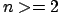and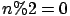):
int i=0;
register double at,bt,st,s=0.0;
while (i<n)
{
at=a[i  ]; bt=b[i  ]; st=at*bt; s+=st;
at=a[i+1]; bt=b[i+1]; st=at*bt; s+=st;
i+=2;
}

To reduce computation time you can re-order the instruction inside the main loop:
int i=0;
register double at,bt,st,s=0.0;
n-=2;
bt=b[i];
while (i<n)
{
at=a[i  ]; st=at*bt; bt=b[i+1]; s+=st;
at=a[i+1]; st=at*bt; bt=b[i+3]; s+=st;
i+=2;
}
s+= a[i  ] * bt    +
a[i+1] * b[i+1];

The second code is faster because it allows parallel execution of instructions. While the arithmetic-unit of the processor is busy computing "st=at*bt" the memory-access-unit of the processor can already performs "bt=b[i+1]". This is called "parallel execution" because the two units are working in parallel. Unfortunately, in our example, the next instruction (after "bt=b[i+1]") is "s+=st". To execute this instruction we must wait until the multiplication "st=at*bt" is finished, loosing some precious time. In other words, there is a "dependency link" between the instructions "st=at*bt" and "s+=st". We should re-order the instructions to remove those "dependency link" to increase parallel execution.

• Let's consider again the unrolled dot product:
int i=n>>3, j=0, k=n&7;
double s=0.0;
while (i--)
{
s+=a[j  ]*b[j  ];
s+=a[j+1]*b[j+1];
s+=a[j+2]*b[j+2];
s+=a[j+3]*b[j+3];
s+=a[j+4]*b[j+4];
s+=a[j+5]*b[j+5];
s+=a[j+6]*b[j+6];
s+=a[j+7]*b[j+7];
j+=8;
}
switch(k)
{
case 7: s+=a[j]*b[j]; j++;
case 6: s+=a[j]*b[j]; j++;
case 5: s+=a[j]*b[j]; j++;
case 4: s+=a[j]*b[j]; j++;
case 3: s+=a[j]*b[j]; j++;
case 2: s+=a[j]*b[j]; j++;
case 1: s+=a[j]*b[j]; j++;
}

There are different ways to implement this "switch instruction" in assembler:
1. CHOICE_1:
                k=n&7;
goto JUMP_TABLE[k];
JUMP_TABLE:  s+=a[j]*b[j]; j++;
JUMP_TABLE:  s+=a[j]*b[j]; j++;
JUMP_TABLE:  s+=a[j]*b[j]; j++;
JUMP_TABLE:  s+=a[j]*b[j]; j++;
JUMP_TABLE:  s+=a[j]*b[j]; j++;
JUMP_TABLE:  s+=a[j]*b[j]; j++;
JUMP_TABLE:  s+=a[j]*b[j]; j++;
JUMP_TABLE:

Note that the C code used here will not actually compile: It's just to have a general idea of what is possible in assembler.
2. CHOICE_2:
k=n&7;
if (k)
{
s+=a[j]*b[j]; j++; k--;
if (k)
{
s+=a[j]*b[j]; j++; k--;
if (k)
{
s+=a[j]*b[j]; j++; k--;
if (k)
{
s+=a[j]*b[j]; j++; k--;
if (k)
{
s+=a[j]*b[j]; j++; k--;
if (k)
{
s+=a[j]*b[j]; j++; k--;
if (k)
{
s+=a[j]*b[j]; j++;
}
}
}
}
}
}
}

3. CHOICE_3:
k=n&7;
if (k)
{
s+=a[j]*b[j];
if (k>3)
{
s+=a[j+1]*b[j+1];
s+=a[j+2]*b[j+2];
s+=a[j+3]*b[j+3];
if (k>5)
{
s+=a[j+4]*b[j+4];
s+=a[j+5]*b[j+5];
if (k==7) s+=a[j+6]*b[j+6];
} else
{
if (k==5) s+=a[j+4]*b[j+4];
}
} else
{
if (k==3)
{
s+=a[j+1]*b[j+1];
s+=a[j+2]*b[j+2];
} else
{
if (k==2)
{
s+=a[j+1]*b[j+1];
}
}
}
j+=k;
}

4. CHOICE_4:
                k=n&7;
double ss=0.0;
goto JUMP_TABLE[k];
JUMP_TABLE:  s +=a[j+6]*b[j+6];
JUMP_TABLE:  ss+=a[j+5]*b[j+5];
JUMP_TABLE:  s +=a[j+4]*b[j+4];
JUMP_TABLE:  ss+=a[j+3]*b[j+3];
JUMP_TABLE:  s +=a[j+2]*b[j+2];
JUMP_TABLE:  ss+=a[j+1]*b[j+1];
JUMP_TABLE:  s +=a[j  ]*b[j];
j+=k;
s+=ss;
JUMP_TABLE:

All the compilers will use either CHOICE_1 or CHOICE_2. In particular, CHOICE_1 *seems* very promising because the processor has no test (no "if" instruction) to perform.

As previously mentioned, the processor is always trying to execute instructions in parallel. This means that while it is processing the current instruction it's already preparing and executing instruction "ahead". When the processor sees a GOTO or JUMP it should be able to guess where it will go using a technique called "branch prediction". A correct guess allows to prepare "ahead" the execution of instructions. A wrong guess will cause a major stall in the pipeline of instructions. Recovering from this pipeline stall is very time-consuming.

CHOICE_3 is superior to CHOICE_2 because it involves less tests. CHOICE_4 is superior to CHOICE_1 because there are less dependency between the instructions. CHOICE_3 and CHOICE_4 were both implemented. Benchmark results indicates that CHOICE_3 is the fastest. CHOICE_3 was thus thoroughly used inside the library. It appears that the only jump performed in CHOICE_4 is harder to predict than all the small jumps performed in CHOICE_3.

• Variables stored in memory are slow to access. This is why there are two levels of cache on x86 processors. The following table summarizes the time required to read data depending on their location:

Location of Data Read time
L1 < 3 nS
L2 < 10 nS
RAM  <100 nS assuming no page page misses plus possible delays to write back a dirty cache line
Disk 10+ milliseconds
Network Disk 100 mS to tens of seconds

The objective of the Prefetch instructions is to load in advance some data in the L1 or L2 cache to decrease the time needed later to read these data. The Prefetch instruction takes as parameter a memory address. The data at this address are copied into the cache. This parameter is difficult to tune. Some preliminary results show that the usage of the Prefetch instruction inside the SDOT, DDOT, SAXPY, DAXPY functions did not lead to any speed increase (on the constrary). I think that more tuning of the Prefetch parameters should be performed. Prefetch instructions are thus currently not used.

# Experimental results

All the tests were performed on the same computer: an INTEL CENTRINO 1.7 GHz with 1GB RAM (DELL inspiron 8600).

All the code optimizations were validated against other strategies using the test benchmark described in this section.

Table 1 displays the time needed (in milliseconds), to process 5000000 calls to the SDOT, DDOT, SAXPY, DAXPY functions on vectors of dimension 200. The vectors are filled with random numbers in [-500 500]. The percentage in the last column shows the relative time compared to the time of the first column.
function
 Standard C code compiled with MVS.NET
 Standard C code compiled with Intel compiler 7.1 on Windows XP
 Standard C code compiled with GCC_3.4 on Linux
 miniSSEL1BLAS Library
SDOT 2984 3217 2964 867 (29.05 %)
DDOT 2938 3130 2963 1501 (51.09 %)
SAXPY 2626 3192 3305 929 (35.38 %)
DAXPY 2666 3173 3290 1484 (55.66 %)
Table 1: Timing results (vector dimension=200; 5000000 calls)

The speed of the library is independent of the compiler and of the operating system since it's written directly in assembler. This is why the performance of the library is reported only once for all compilers and operating systems.

On this special benchmark, The INTEL compiler and the GCC meta-compiler are slower than the Visual Studio .NET compiler. Indeed the assembly code generated by the Visual Studio .NET compiler is from far cleaner than the other assembly code generated by the other compilers.

The SDOT and DDOT functions are close to the theoretical lower boundary of, respectively, 25 % and 50% of running time compared to the standard C implementation. The SAXPY and DAXPY functions are a little bit slower than the SDOT and DDOT functions because they are accessing memory more heavily. Table 2 shows other results with larger vector dimensions.
function
 Standard C code compiled with MVS.NET
 Standard C code compiled with Intel compiler 7.1 on Windows XP
 Standard C code compiled with GCC_3.4 on Linux
 miniSSEL1BLAS Library
SDOT 2480 2823 2683 947 (38.19 %)
DDOT 2549 2784 2759 1896 (74.38 %)
SAXPY 2425 2707 2888 1120 (46.19 %)
DAXPY 2826 2965 3054 2255 (79.79 %)
Table 2: Timing results (vector dimension=20000; 50000 calls)

Table 2 indicates that, for larger vector sizes, the memory bottleneck becomes more prominent. Obviously, a correct Prefechtching should help in this case.

## Annexe

The standard C code implementation of SDOT,DDOT,SAXPY,DAXPY that is used in the experimental results is:
double  ddot_C(int n, double *x,double *y)
{ double s=0.0; while (n--)     { s+=*(x++) * *(y++); }; return s; }

float   sdot_C(int n, float  *x,float  *y)
{ float  s=0.0; while (n--)     { s+=*(x++) * *(y++); }; return s; }

void   saxpy_C(int n, float   a,float  *x, float  *y)
{               while (n--)  *(x++)+=  a    * *(y++); }

void   daxpy_C(int n, double  a,double *x, double *y)
{               while (n--)  *(x++)+=  a    * *(y++); }


# User's guide for the FORTRAN interface to the library

There is one example inside the ZIP file that demonstrates the usage of the FORTRAN interface to the library. The example is for a UNIX fortran compiler (f77/g77) and is makefile-based. The miniSSEL1BLAS library is composed by only two files: miniSSEL1BLAS.cpp and miniSSEL1BLAS.h. Basically, to use the library in your own project, you must generate an object file "miniSSEL1BLAS.o" using the command:
g++ -O -c miniSSEL1BLAS.cpp

Thereafter, you can simply add the object file " miniSSEL1BLAS.o" to the list of FORTRAN ".f" file that are inside your project. Usually, you obtain something like:
f77 -o <name_of_the_executable> <list_of_fortran_.f_files> miniSSEL1BLAS.o

The FORTRAN interface implements the following FORTRAN functions:

integer          function   hasSSE()                 // out=1 if SSE  supported, out=0 otherwise

integer          function   hasSSE2()                // out=1 if SSE2 supported, out=0 otherwise

subroutine scopy    (n,  sx,sy)     // y[i]=x[i]        for_all_i
subroutine dcopy    (n,  dx,dy)

subroutine saxpy    (n,sa,sx,sy)    // y[i]=a*x[i]+y[i] for_all_i
subroutine saxpyUA  (n,sa,sx,sy)
subroutine daxpy    (n,da,dx,dy)
subroutine daxpyUA  (n,da,dx,dy)

subroutine sscale   (n,sa,sx)       // x[i]=a*x[i]      for_all_i
subroutine dscale   (n,da,dx)
subroutine sscaleUA (n,sa,sx)
subroutine dscaleUA (n,da,dx)

real             function   sdot     (n,   sx,sy)   // out = sum_over_i x[i]*y[i]
real             function   sdotUA   (n,   sx,sy)
double precision function   ddot     (n,   dx,dy)
double precision function   ddotUA   (n,   dx,dy)

real             function   ssquare  (n,   sx)      // out = sum_over_i x[i]**2
real             function   ssquareUA(n,   sx)
double precision function   dsquare  (n,   dx)
double precision function   dsquareUA(n,   dx)

real             function   snrm2    (n,   sx)      // out = sqrt(square(n,x)/n)
real             function   snrm2UA  (n,   sx)
double precision function   dnrm2    (n,   dx)
double precision function   dnrm2UA  (n,   dx)

real             function   sasum    (n,   sx)      // out = sum_over_i fabs(x[i])
real             function   sasumUA  (n,   sx)
double precision function   dasum    (n,   dx)
double precision function   dasumUA  (n,   dx)

real             function   smin     (n,   sx)      // out = min_for_all_i x[i]
real             function   sminUA   (n,   sx)
double precision function   dmin     (n,   dx)
double precision function   dminUA   (n,   dx)

real             function   smax     (n,   sx)      // out = max_for_all_i x[i]
real             function   smaxUA   (n,   sx)
double precision function   dmax     (n,   dx)
double precision function   dmaxUA   (n,   dx)

subroutine sswap    (n,   sx,sy)   // SWAP(x[i],y[i])  for_all_i
subroutine dswap    (n,   dx,dy)
subroutine sset     (n,sa,sx)      // x[i]=a           for_all_i
subroutine dset     (n,da,dx)

The data type of the arguments are:
    integer n
real sx(*),sy(*),sa,sc,ss
double precision dx(*),dy(*),da,dc,ds

Six additional functions/subroutines are provided in order to meet the L1 BLAS requirements. WARNING: these functions are NOT optimized and are thus relatively slow:
    integer function   suiamax( n,sx)             // out = index i of the maximum of fabs(x[i])
integer function   duiamax( n,dx)
subroutine srot   (sx,sy,sc,ss)       // apply a 2D rotation(c,s) on all the 2D points (x[i],[i])
subroutine drot   (dx,dy,dc,ds)
subroutine srotg  (sa,sb,sc,ss)       // compute a 2D rotation
subroutine drotg  (da,db,dc,ds)

The data type of the arguments are:
        integer n
real sx(*),sy(*)sa,sb,sc,ss
double precision dx(*),dy(*),da,db,dc,ds

Before using the Level 1 BLAS functions, you should check if the SSE set (or SSE2 set) of instructions is supported by your processor. The library provides the function "hasSSE" (and the function "hasSSE2") that returns one if SSE (respectively SSE2) is suppported. Operations in single precision require SSE support only. Operations in double precision require SSE2 support.

All the vector arrays given as parameters to the LEVEL 1 BLAS FUNCTIONS must start on a memory address that is a multiple of 16 (this is called 16-byte alignment). By default, fortran compilers (g77) always allocates arrays with correct alignment. This means that you can easily do:
int offset,i,n
real              sx(100), sy(100), sr
double precision  dx(100), dy(100), dr

sr=sdot(n,sx,sy)
dr=ddot(n,dx,dy)

However, the next example will work only if ((offset-1)%4)=0 ( The "%" stands for the modulo operation):
sdot(n,sx(offset),sy(offset+i*4))

The next example will only work if ((offset-1)%2)=0 :
ddot(n,dx(offset),dy(offset+i*2))

If you need to work on UnAligned arrays, you can use the LEVEL 1 BLAS FUNCTIONS that have the UA suffix. The UA suffix inside the name of the function stands for "UnAligned" memory address. The UA version of the function is slower but the vector arrays parameters can be un-aligned. However the un-alignement must be the same for x and for y. In other word, the following example works for any value of the variable "offset":
int offset,i,n
real s(*)
double precision d(*)

sdotUA(n,s(offset),s(offset+i*4))
ddotUA(n,d(offset),d(offset+i*2))

The functions scopy, dcopy, sswap, dswap, sset, dset, suiamax, duiamax, srot, drot, srotg, drotg have no UnAligned equivalent. The vector array parameters given to these functions can be UnAligned.

# User's guide for the C++ interface to the library

The library is composed by two files: miniSSEL1BLAS.cpp and miniSSEL1BLAS.h. These are the only 2 files that you need to include into your own projects to be able to use the library.
There are two examples to demonstrate the usage of the C++ interface to the library:
1. a Visual Studio .NET example (.sln)
2. a GCC example (makefile based)
The examples are the benchmarks used in section 1.2.

Before using the Level1 BLAS functions, you should check if the SSE set (or SSE2 set) of instructions is supported by your processor. The library provides the global boolean variable " hasSSE" (and the global variable "hasSSE2") that is true if SSE (respectively SSE2) is supported. Operations on float require SSE support only. Operations on double require SSE2 support.

All the vector arrays given as parameters to the LEVEL 1 BLAS FUNCTIONS must start on a memory address that is a multiple of 16 (this is called 16-byte alignment). When using the classical C "malloc" function, only a 8-byte alignment is assured. You must use the following memory allocations functions to be assured of correct alignment (these functions are part of the miniSSEL1BLAS library):
void *mallocAligned(unsigned long n);   // malloc aligned on 16 byte boundaries
void freeAligned(void *t);              // free the aligned allocation

Let's consider this small C code:
int i= ...any number...
float *f=(float*)mallocAligned(...any number...),
*fA=f+i*4;
double *d=(double*)mallocAligned(...any number...),
*dA=d+i*2;

You can use the vector arrays f,fA,d,dA inside the Level1 BLAS functions because all these arrays are correctly aligned.

The LEVEL 1 BLAS functions are available as standard C++ functions as defined below.
    float  dot     (int n,            float  *x, float  *y);  // out = sum_over_i x[i]*y[i]
double dot     (int n,            double *x, double *y);
void   axpy    (int n, float   a, float  *x, float  *y);  // y[i]+=a*x[i]  for_all_i
void   axpy    (int n, double  a, double *x, double *y);
void   vcopy   (int n,            float  *x, float  *y);  // y[i]=x[i]      for_all_i
void   vcopy   (int n,            double *x, double *y);
void   vscale  (int n, float   a, float  *x);             // x[i]*=a        for_all_i
void   vscale  (int n, double  a, double *x);
float  asum    (int n,            float  *x);             // out = sum_over_i fabs(x[i])
double asum    (int n,            double *x);
float  square  (int n,            float  *x);             // out = sum_over_i x[i]**2
double square  (int n,            double *x);
float  nrm2    (int n,            float  *x);             // out = sqrt(square(n,x)/n)
double nrm2    (int n,            double *x);
float  vmin    (int n,            float  *x);             // out = min_for_all_i x[i]
double vmin    (int n,            double *x);
float  vmax    (int n,            float  *x);             // out = max_for_all_i x[i]
double vmax    (int n,            double *x);
void   vswap   (int n,            float  *x, float  *y);  // SWAP(x[i],y[i])  for_all_i
void   vswap   (int n,            double *x, double *y);
void   vset    (int n, float   a, float  *x);             // x[i]=a           for_all_i
void   vset    (int n, double  a, double *x);

Six additional functions/subroutines are provided in order to meet the L1 BLAS requirements. WARNING: these functions are NOT optimized and thus are relatively slow:
  int  uiamax(int  n, double *x);                                 // out = index i of the maximum of fabs(x[i])
int  uiamax(int  n, float  *x);
void rot   (int *n, float  *x, float  *y, float   c,float   s); // apply a 2D rotation(c,s) on all the 2D points (x[i],[i])
void rot   (int *n, double *x, double *y, double  c,double  s);
void rotg  (        float  *a, float  *b, float  *c,float  *s); // compute a 2D rotation
void rotg  (        double *a, double *b, double *c,double *s);

The usage is straightforward as soon as the vector arrays x and y are correctly aligned. If the arrays x and y are not correctly aligned, you can still use:
    float  dotUA   (int n,            float  *x, float  *y);
double dotUA   (int n,            double *x, double *y);
void   axpyUA  (int n, float   a, float  *x, float  *y);
void   axpyUA  (int n, double  a, double *x, double *y);
void   vscaleUA(int n, float   a, float  *x);
void   vscaleUA(int n, double  a, double *x);
float  asumUA  (int n,            float  *x);
double asumUA  (int n,            double *x);
float  squareUA(int n,            float  *x);
double squareUA(int n,            double *x);
float  nrm2UA  (int n,            float  *x);
double nrm2UA  (int n,            double *x);
float  vminUA  (int n,            float  *x);
double vminUA  (int n,            double *x);
float  vmaxUA  (int n,            float  *x);
double vmaxUA  (int n,            double *x);

The suffix "UA" stands for "Un-Aligned". The UnAligned versions of the functions is slower. Furthermore, the un-alignement must be the same for x and for y: We must have (x%16)=(y%16). The "%" sign is the modulo operator. In other words, we can have:
int i;
float  *f=(float *)malloc(...);       // f is not aligned
double *d=(double*)malloc(...);       // d is not aligned
...
sdotUA(n,f,f+i*4);       // f is not aligned
ddotUA(n,d,d+i*2);       // d is not aligned

In opposition, we cannot have:
sdot(n,f,f+i*4);         // f is not aligned
ddot(n,d,d+i*2);         // d is not aligned

The functions vcopy, vswap, vset, uiamax, rot, rotg have no UnAligned equivalent. The vector array parameters given to these functions can be UnAligned.

Some LEVEL 1 BLAS functions are also available as C++ MACRO's:
unsigned int n;
float  *xf,*yf,af,rf;  // xf,yf are correctly aligned.
double *xd,*yd,ad,rd;  // xd,yd are correctly aligned.

SDOT_SSE (A,n,   xf,yf,rf)    // rf = sum_over_i xf[i]*yf[i]
DDOT_SSE2(A,n,   xd,yd,rd)    // rd = sum_over_i xd[i]*yd[i]
SAXPY_SSE (A,n,af,xf,yf)       // yf[i]+=af*xf[i]  for_all_i
DAXPY_SSE2(A,n,ad,xd,yd)       // yd[i]+=ad*xd[i]  for_all_i
SSCALE_SSE (A,n,af,xf)          // xf[i]*=af        for_all_i
DSCALE_SSE2(A,n,ad,xd)          // xd[i]*=ad        for_all_i
SSQUARE_SSE (A,n,   xf,rf)       // rf = sum_over_i xf[i]**2
DSQUARE_SSE2(A,n,   xd,rd)       // rd = sum_over_i xd[i]**2
SASUM_SSE (A,n,   xd,rd)       // rf = sum_over_i fabs(xf[i])
DASUM_SSE2(A,n,   xf,rd)       // rd = sum_over_i fabs(xd[i])
SMIN_SSE (A,n,   xf,rf)       // rf = min_for_all_i xf[i]
DMIN_SSE2(A,n,   xd,rd)       // rd = min_for_all_i xd[i]
SMAX_SSE (A,n,   xf,rf)       // rf = max_for_all_i xf[i]
DMAX_SSE2(A,n,   xd,rd)       // rd = max_for_all_i xd[i]

float  *xfu,*yfu,af;  // xfu,yfu can be Un-Aligned.
double *xdu,    ,ad;  // xdu     can be Un-Aligned.

MEMSWAP_MMX (A,n,   xfu,yfu)     // SWAP(xf[i],yf[i])  for_all_i
SMEMSET_MMX (A,n,af,xfu)         // xf[i]=af           for_all_i
DMEMSET_MMX (A,n,ad,xdu)         // xd[i]=ad           for_all_i

The arguments given to the MACRO's must exactly be of the type specified above. The vector arrays xf,yf,xd,yd must be correctly aligned. Let's now look at the first argument (in the examples above, it's "A"). If you try to compile the following code, the compiler will complain about multiple definition of the same symbols:
...
float  *x1,*y1,r1,
*x2,*y2,r2;
...
SDOT_SSE(A,n,x1,y1,r1);
SDOT_SSE(A,n,x2,y2,r2);
...

The following code compiles without any problem:
...
SDOT_SSE(A,n,x1,y1,r1);
SDOT_SSE(B,n,x2,y2,r2);
...

The first argument of the MACRO must be different at each inclusion of the MACRO inside the same file. As you can see, the usage of the MACRO version of the functions is slightly more complex. On the other hand, you can remove all the function call overheads that are quite time-consuming on big loops.

# What processor can I use?

All the functions (except vcopy, vset and vswap) are using either SSE or SSE2 intructions. Operations on floats are performed using SSE instruction sets only. Operations on doubles are performed using SSE2 instructions.

SSE support is included in:
• Intel® Pentium III and above
• AMD's 3Dnow!™ Professional technology, which is supported in
• AMD Athlon XP
• AMD Athlon MP
• mobile AMD Athlon 4
• Model 7 AMD Duron™ processors.
• AMD opteron, AMD Athlon 64 and above
The oldest AMD processor supporting SSE is an AMD Athlon XP 1500+ at a frequency of 1333 MHz.
SSE2 support is included in:
• Intel® Pentium 4, Intel® Pentium M
• AMD opteron, AMD Athlon 64 and above

# References

To have some good guidelines on how to write optimized loops containing conditional branches, see the article about the D-loop pattern. I really think that every programmer can gain some clever insight through this article. That's a must-read! The article is available here. A local copy of the article is here: d-loop.pdf

The famous BLAS library: BLAS (Basic Linear Algebra Subprograms): http://www.netlib.org/blas/
Quick-Reference to all the BLAS functions: blasqr.pdf.

Here are some "improved version" of the standard BLAS library:
• ATLAS: Automatically Tuned Linear Algebra Software:
http://math-atlas.sourceforge.net/

When you install ATLAS on your computer, it tries to guess what's the optimum loop-unrolling. It also tries different orders for the C instructions. This last option is somewhat redundant with a good compiler. The performances of ATLAS are reported to be very close to the optimum theoretical performances of the processor when using the classical set (no SSE, no SSE2) of assembly-level instructions.

Recently, some x86 assembler code was included inside ATLAS. However, the coders from ATLAS don't want to bother the users with such thing like "memory alignment". In most cases, this prevents them to write SSE-optimized functions. Performance of the SDOT,DDOT,SAXPY,DAXPY functions are thus low compared to the miniSSEL1BLAS library.

• AMD Core Math Library (ACML)
http://developer.amd.com/acml.aspx

AMD is providing an optimized Matrix processing library for all its processors. This is a commercial library. Source code is not available. However free download for evaluation purposes for Windows and Linux is available. If you desire to distribute any part of this library inside a commercial product, you shall enter into a separate written agreement with AMD.

• INTEL IPP: Intel Integrated Performance Primitives
http://www.intel.com/cd/software/products/asmo-na/eng/perflib/ipp/index.htm

INTEL is providing an optimized Matrix processing library for its pentium processors. This is a commercial library. Source code is not available. However free download for non-commercial
applications (and only for the Linux OS) is available.

• LFMat: LFMat is a templatized open source C++ matrix library with storage-compatible and assembler specializations for 3Dnow!, SSE and SSE2.
http://lfmat.sourceforge.net/

The library should give better performances than the ATLAS library since it is using SSE/SSE2 sets of instructions. It is however still in beta currently (August 2005).

• Blitz++: Scientific computing C++ library. Uses template techniques to achieve high performance.
http://www.oonumerics.org/blitz/

Blitz++ uses templates techniques to know at compile-time, for each user loop, the optimal unrolling and the optimal order of the instructions. The information needed to optimize the loops are, basically, the exact size of the vectors and the exact size of the matrices involved. These sizes are fixed and must be given at compilation time. If this is not a problem for you, Blitz++ should give even better performances than ATLAS.

• miniSSEL1BLAS library: implements only LEVEL 1 BLAS functions on x86 processor with SSE/SSE2 support.
http://www.applied-mathematics.net/miniSSEL1BLAS/miniSSEL1BLAS.html

Many assembler level optimizations have been performed to ensure that peak performances are attained. It's currently the only library that forces you to use 16-bytes-memory-aligned vector arrays. Thanks to that, it should be faster than any other implementation. And it's free! Available for direct download! Drawback: for some functions, the user MUST use 16-bytes-memory-aligned vector arrays.
Some good overall information on MMX/SSE/SSE2 technology:
Some information about Prefetching for x86:
• James Mc Parlane's Blog: x86 Cache Prefetch:
• Memory Access is the Key To Speed (The table about access time for the different kind of memory is coming from this site).
Some part of the miniSSEL1BLAS library is inspired by code found in the GnuRadio: http://www.gnu.org/software/gnuradio/
These guys know how to code!

I am usually coding in assembler using Intel MASM style. To know how to convert MASM style assembler code to GCC-gnu style, see Brennan's Guide to Inline GNU Assembly: http://www.delorie.com/djgpp/doc/brennan/brennan_att_inline_djgpp.html (local copy here).

Assembler implementation of a very efficient memcpy function.

Some information about binary storage inside memory of floating point numbers: float-ieee754.pdf